﻿ 基于图勾勒的图链路预测方法
«上一篇文章快速检索 高级检索

 智能系统学报2019, Vol. 14Issue (4): 761-768  DOI: 10.11992/tis.2018060070

### 引用本文YOU Jie, LI Jin, ZHANG Sai, et al. Graph sketches-based link prediction over graph data[J]. CAAI Transactions on Intelligent Systems, 2019, 14(4): 761-768. DOI: 10.11992/tis.201806007.### 文章历史

1. 云南大学 软件学院，云南 昆明 650091;
2. 云南省软件工程重点实验室，云南 昆明 650091

Graph sketches-based link prediction over graph data
YOU Jie 1, LI Jin 1,2, ZHANG Sai 1, LI Ting 1
1. School of Software, Yunnan University, Kunming 650091, China;
2. Key Laboratory in Software Engineering of Yunnan Province, Kunming 650091, China
Abstract: The high computational complexity of existing link prediction algorithms makes them unsuitable for link prediction on large-scale graphs. To solve this problem, we propose a novel link prediction approach that involves combining the existing link prediction approaches with graph sketch approximation. Our proposed approach reduces the computation complexity of link prediction from $O\left( {{n^3}} \right)$ to $O\left( {{n^2}{k^2}{\rm{lo{g}}^2}n} \right)$ Furthermore, to enhance the efficiency of our approach; we also provide a parallel link prediction algorithm, which is implemented on the parallel computing framework Apache Spark. Finally, we conducted extensive experiments on a real network dataset to test the validation and efficiency of our approach. The experimental results indicate that our methods can effectively improve the efficiency of link prediction while guaranteeing prediction accuracy as well.
Key words: graph data    algorithm complexity    link-prediction    graph sketches    nodes similarity    parallel computing    Apache Spark

1 背景知识 1.1 链路预测

 $S_{xy}^{{\rm{CN}}} = \left| {\varGamma \left( x \right) \cap \varGamma \left( y \right)} \right|$ (1)

 $S_{xy}^{{\rm{AA}}} = \mathop \sum \limits_{z \in \varGamma \left( x \right) \cap \varGamma \left( y \right)} \frac{1}{{\log\left( {{d_z}} \right)}}$ (2)

 $S_{xy}^{\rm{{RA}}} = \mathop \sum \limits_{z \in \varGamma \left( x \right) \cap \varGamma \left( y \right)} \frac{1}{{{d_z}}}$ (3)

 ${\rm{AUC}} = \frac{{{n'} + 0.5{n''}}}{n}$ (4)
1.2 图勾勒技术

 ${\rm{ADS}}\left( v \right) = \{ \left( {u, d\left( {v, u} \right)} \right)|r\left( u \right) < K_r^{\rm th}\left( {N\left( {v, u} \right)} \right)\}$ (5)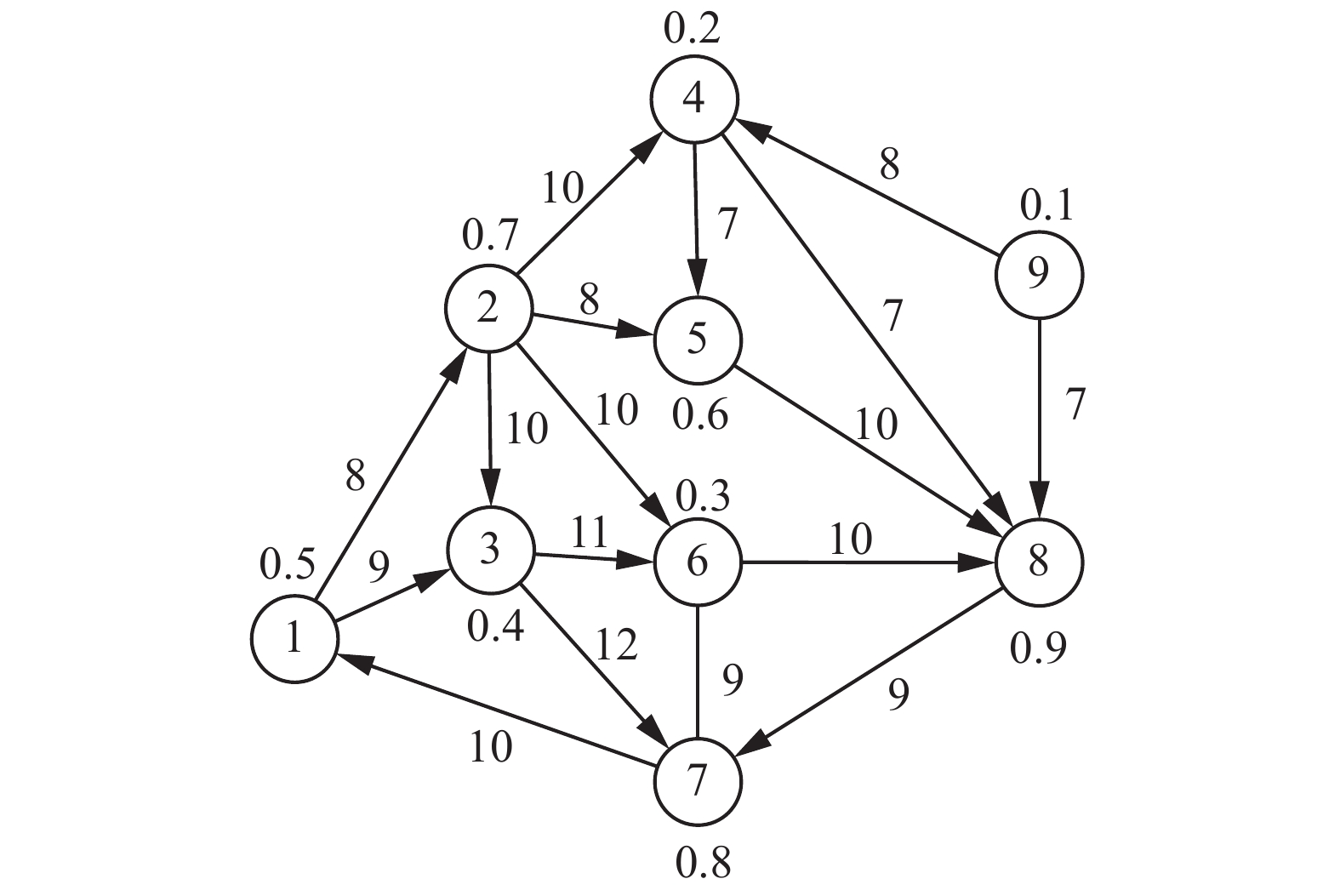Download: 图 1 图的ADS示例 Fig. 1 An illustration of ADS in a graph

 \begin{aligned} {\rm{ADS}}\left( 1 \right) = \{ \left( {1, 0} \right), \left( {2, 8} \right), \left( {3, 9} \right), \left( {4, 18} \right), \left( {6, 18} \right){\rm{\} }}\qquad\quad\\ {\rm{ADS}}\left( 2 \right) = {\text{\{ (2, 0), (5, 8), (4, 10), (6, 10), (3, 10)\} }}\qquad\\ {\rm{ADS}}\left( 5 \right) \!\!=\!\! \{ (5, 0), \!(8, 10), \!(7, 19), \!(6, 28),\! (1, 29),\! (3, 38),\! (4, 47)\} \end{aligned}

2 链路预测方法

 $S_{xy}^{{\rm{ADS - CN}}} = \left| {{\rm{ADS}}{{\left( x \right)}_1} \cap {\rm{ADS}}{{\left( y \right)}_1}} \right|$ (6)

 $S_{xy}^{{\rm{ADS - AA}}} = \mathop \sum \limits_{z \in {\rm{ADS}}{{\left( x \right)}_1} \cap {\rm{ADS}}{{\left( y \right)}_1}} \frac{1}{{{\rm{log}}\left( {{d_z}} \right)}}$ (7)

 $S_{xy}^{{\rm{RA}}} = \mathop \sum \limits_{z \in {\rm{ADS}}{{\left( x \right)}_1} \cap {\rm{ADS}}{{\left( y \right)}_1}} \frac{1}{{{d_z}}}$ (8)

1) 切割边集E为训练集Er和测试集Ep $E = {E_r} \cup {E_p}, {E_r} \cap {E_p} = \text{Ø}$

2) ${\rm{degree}}\left( v \right) \leftarrow$ 求出Er中所有结点的结点度；

$(G' = \left( {V, {E_r}} \right), v \in$ V)

3) ${\rm{ADS}}\left( v \right) \leftarrow$ 根据式(3)构造图 $G'$ 中个结点的；

${\rm{ADS}}(G' = \left( {V, {E_r}} \right), v \in$ V)

//找出训练集中不存在连边的结点对集合

4) $A \leftarrow \left\{ {\left( {v, w} \right)|v, w \in V{\text{且}}\left( {v, w} \right) \in \overline {{E_r}} } \right\}$

5) ${S_{vw}} \leftarrow$ 求出A中所有节点对的预测值；

//得到 $\overline E$ 中所有连边结点对的预测值；

6) ${S_{xy}} \leftarrow \left\{ {S\left( {x, y} \right)|x, y \in V{\text{且}}\left( {x, y} \right) \in \overline E } \right\}$

//得到 ${E_p}$ 中所有连边结点对的预测值；

7) ${S_{uv}} \leftarrow \left\{ {S\left( {u, v} \right)|u, v \in V{\text{且}}\left( {u, v} \right) \in {E_p}} \right\}$

8) ${\rm{for}} \; i \leftarrow 1 \; {\rm{to}}\; n \; {\rm{do}}$

9) a：从 ${S_{uv}}$ 中选出一条边的预测值；

10) $b:{S_{xy}}$ 中选出一条边的预测值；

11) $n'$ ：表示 ${E_p}$ 中的预测值大于 $\overline E$ 中预测值的次数；

12) $n''$ ：表示 ${E_p}$ 中的预测值等于 $\overline E$ 中预测值的次数；

13) ${\rm{if}} \; a > b \;{\rm{do}}$

14) $n' = n' + 1$

//创建边集 $E$ ${\rm{RDD}}$

1) ${\rm{dataRDD \leftarrow sc.textFile(edgesPath}})$

//创建训练集 ${E_r}$ ${\rm{RDD}}$

2) ${\rm{edgestRDD}} \leftarrow {\rm{dataRDD}}$ .takeSample (0.9 * ${ \left( {{\rm{dataRDD.count}}} \right)} )$

//创建测试集 ${E_p}$ ${\rm{RDD}}$

3) ${\rm{edgespRDD}} \leftarrow {\rm{dataRDD.subtract}}\left( {{\rm{edgestRDD}}} \right)$

//找出训练集中不存在连边的结点对集合

4) ${\rm{pairRDD}} \leftarrow {\rm{edgestRDD.cartesian}}\left( {{\rm{edgestRDD}}} \right)$ . ${\rm{subtract}}\left( {{\rm{edgestRDD}}} \right)$

//求出各顶点的邻居节点

5) ${\rm{verticesRDD}} \leftarrow {\rm{edgestRDD.groupByKey}}()$ .map $\left( {{\rm{vertices.nbrs}}} \right)$

6) $g \leftarrow {\rm{Graph}}\left( {{\rm{verticesRDD}}, {\text{ }}{\rm pairRDD}} \right)$ ；//构造图 $g$

//求出各结点的节点度

(7) ${\rm{degreeRDD}} \leftarrow {\rm{verticesRDD.map}}\left( {{\rm{nbrs.size}}} \right)$

//求结点x, y的相似度

8) ${\rm{simRDD}} \leftarrow {\rm{g.triplets.map}}\left( {{\rm{sim}}\left( {{\rm{x, {\text{ }}y.persist}}()} \right)} \right)$

9) ${\rm{simepRDD}} \leftarrow$ 从simRDD筛选 ${E_p}$ 连边的预测值；

10) ${\rm{simeNotRDD}} \leftarrow$ 从simRDD筛选 ${E_P}$ 连边预测值；

11) $a \leftarrow {\rm{simepRDD.takeSample}}\left( {{\rm{true}}, {\text{ }}n} \right)$

3 实验结果 3.1 实验环境设置表 1 实验数据集拓扑结构信息 Tab.1 Experimental dataset topology information

3.2.1 两种算法执行效率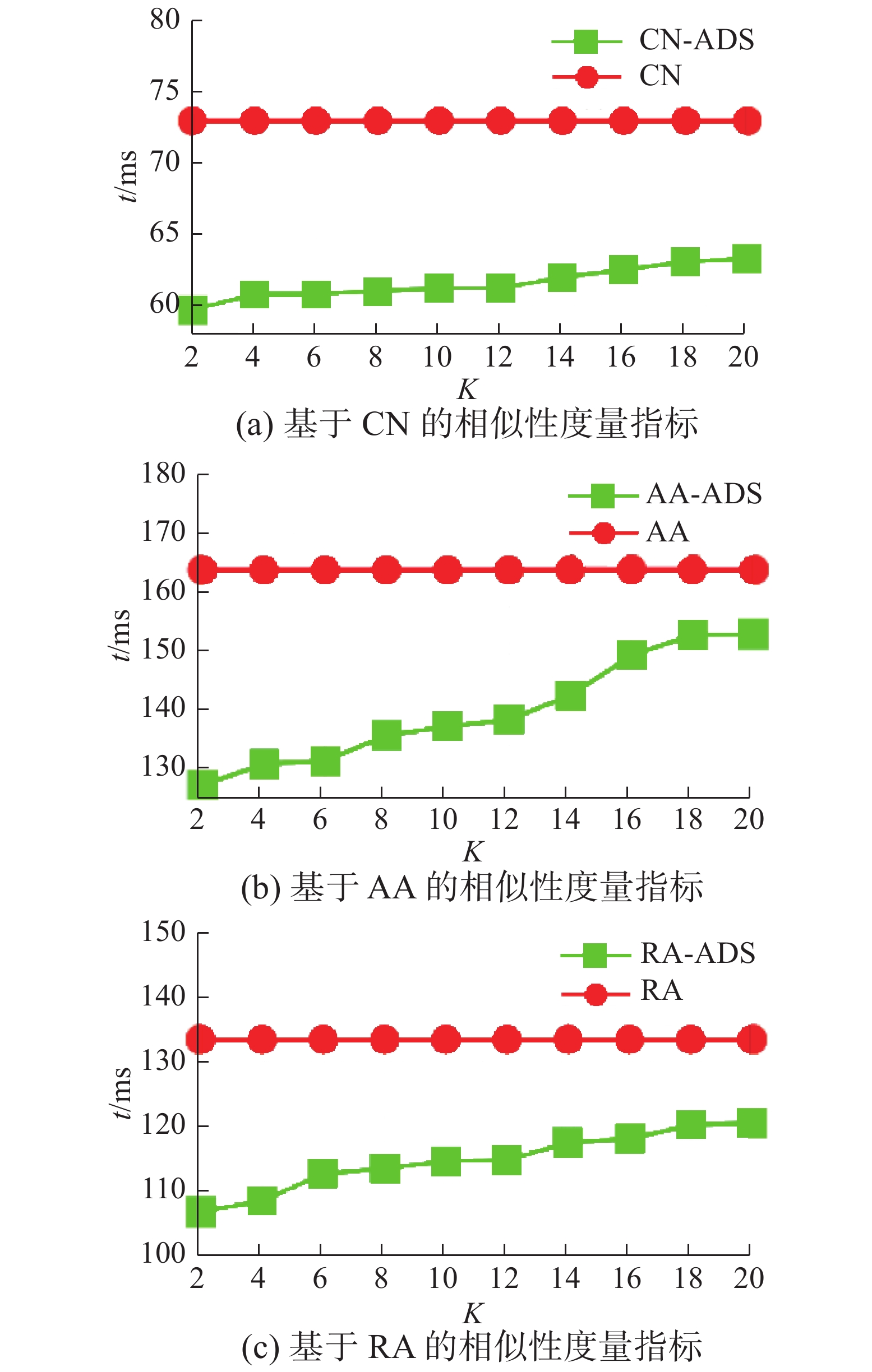Download: 图 2 CN、AA、RA度量指标运行时间对比(USAir97) Fig. 2 CN, AA, RA metrics comparison of run time (USAir97)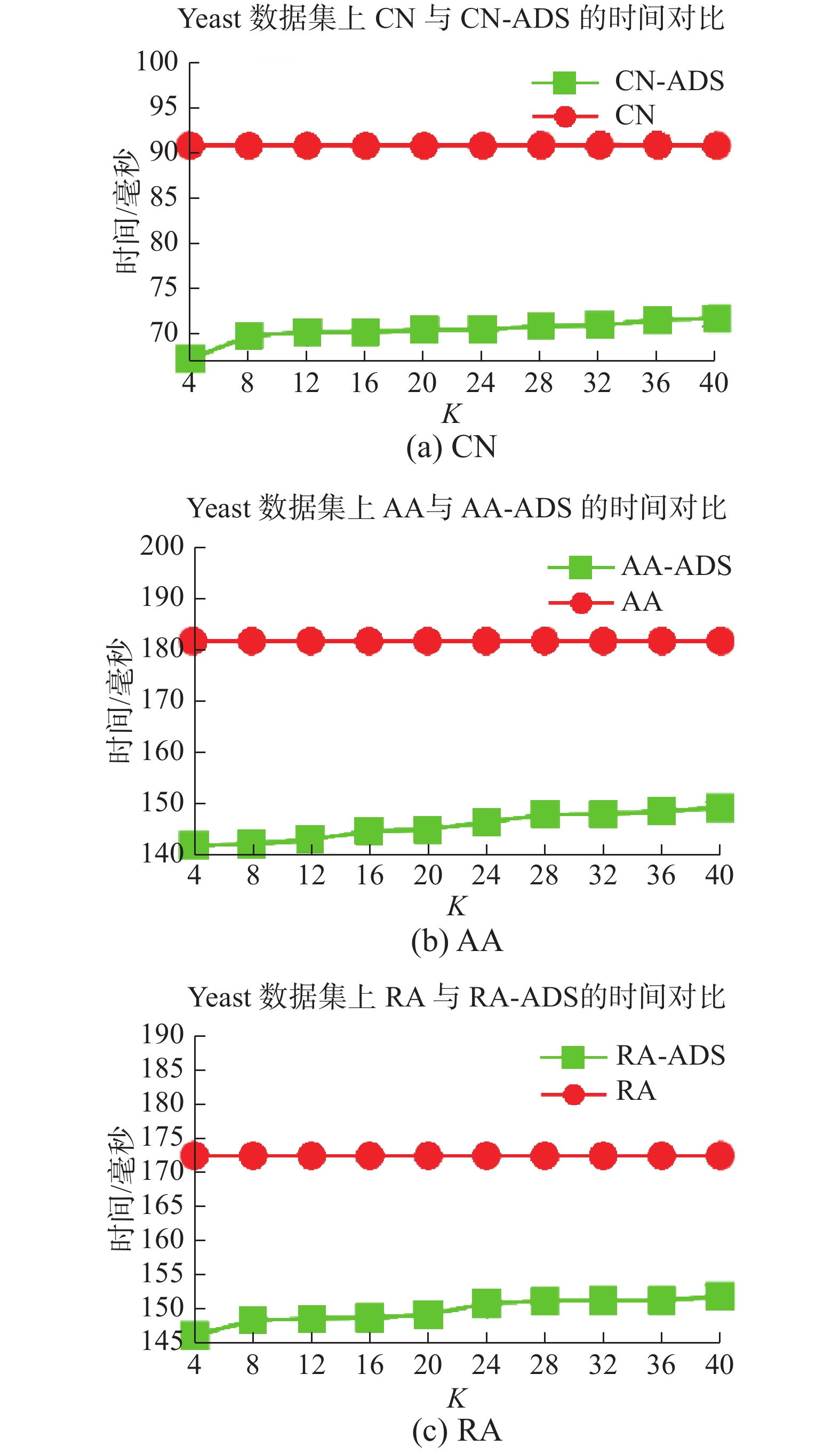Download: 图 3 CN、AA、RA度量指标运行时间对比(Yeast) Fig. 3 CN，AA，RA metrics comparison of run time (Yeast)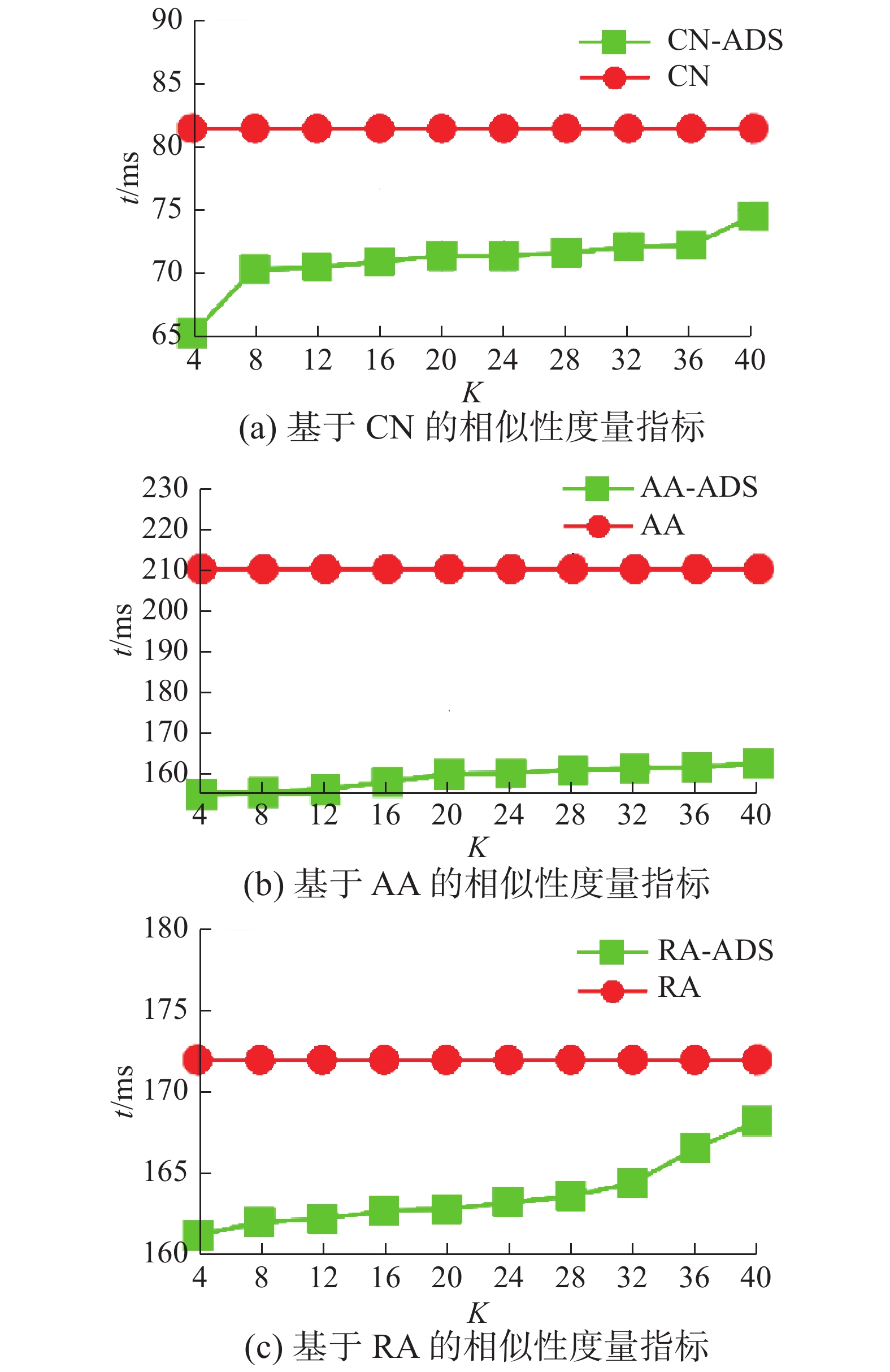Download: 图 4 CN、AA、RA度量指标运行时间对比(Grid) Fig. 4 CN，AA，RA metrics comparison of run time (Grid)
3.2.2 两种算法的预测精度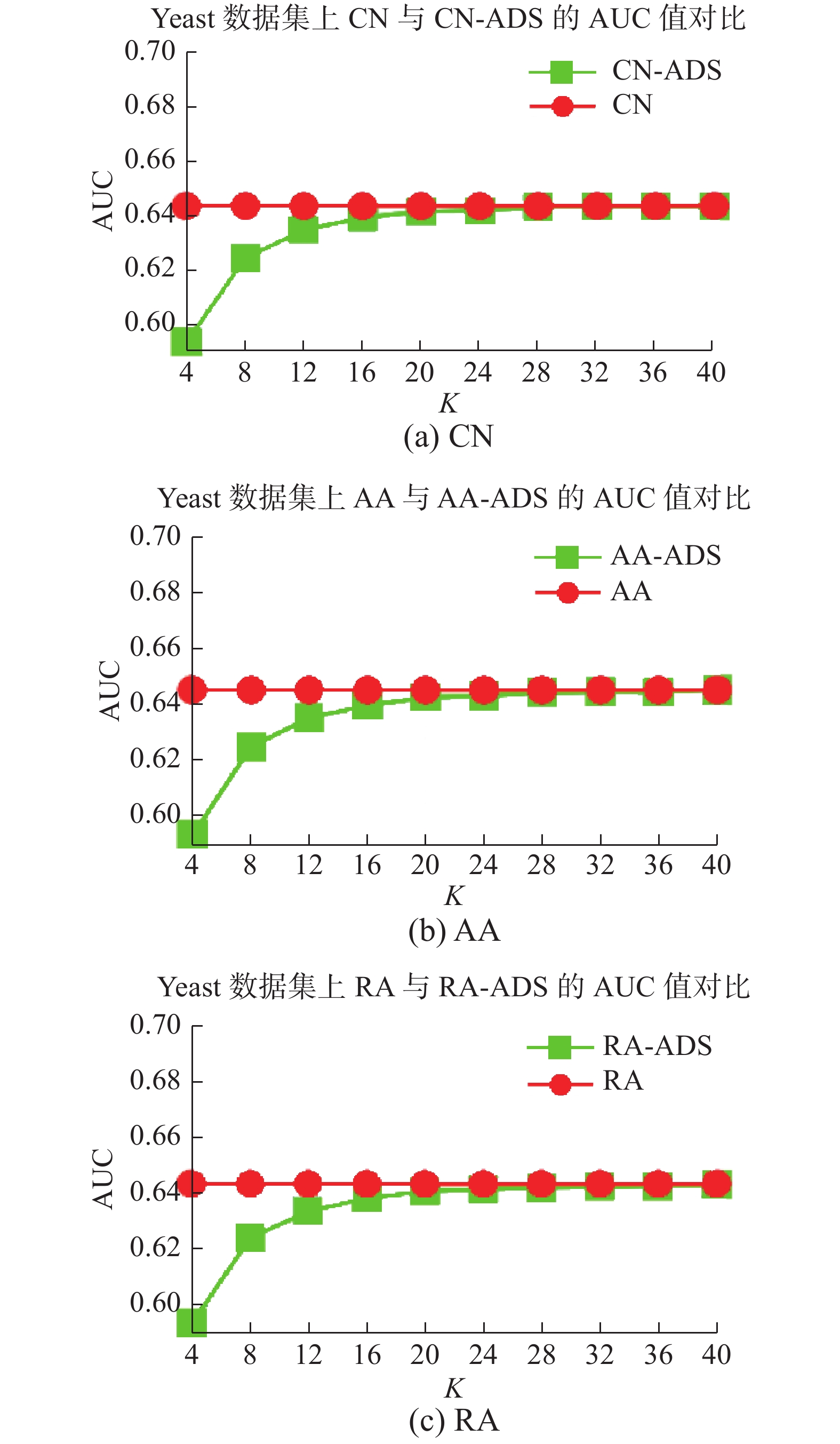Download: 图 6 CN、AA、RA 度量指标 AUC 对比 (Yeast) Fig. 6 Comparison of the CN，AA，RA metrics AUC (Yeast)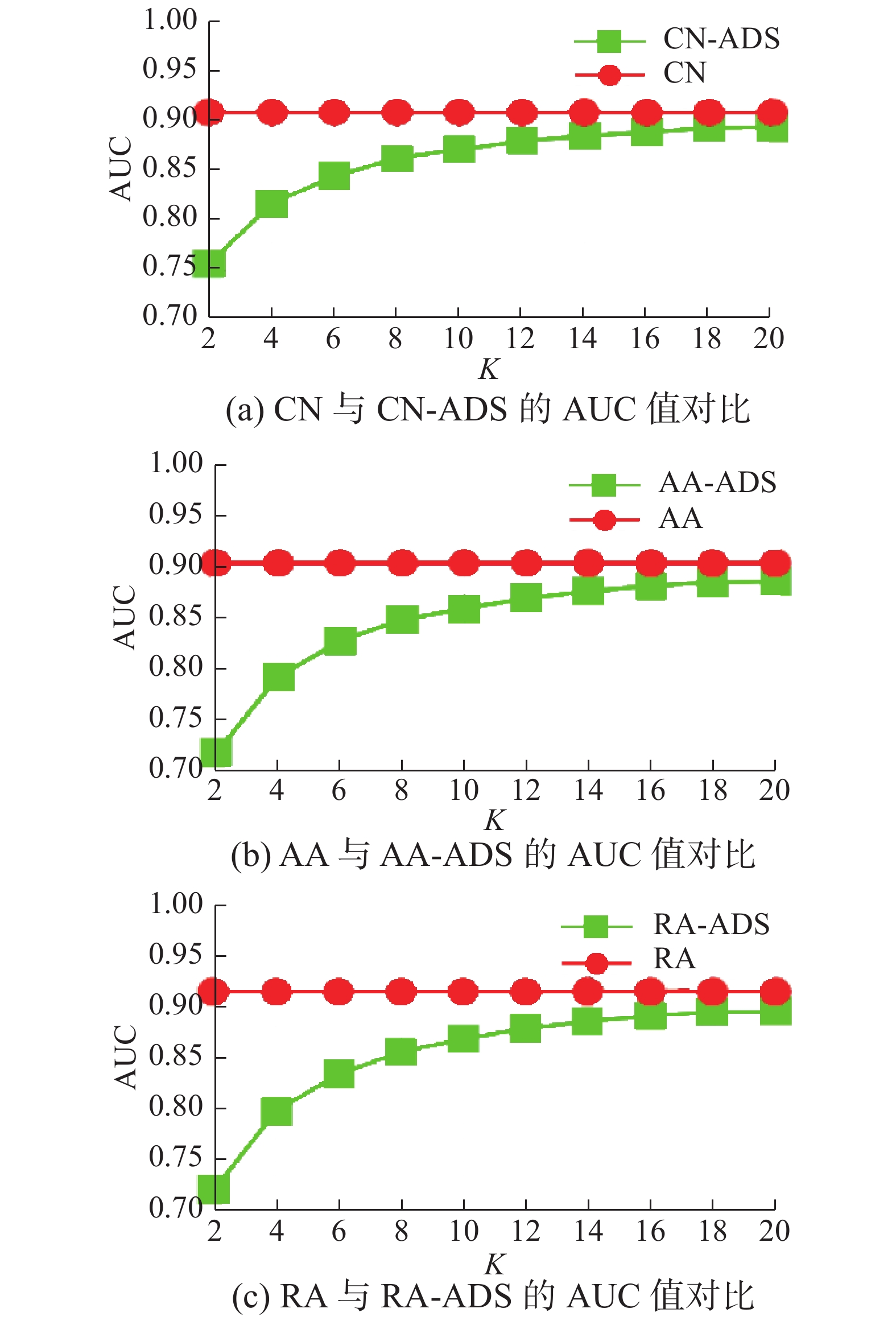Download: 图 5 CN，AA，RA度量指标AUC对比(USAir97) Fig. 5 Comparison of the CN, AA, RA metrics AUC (USAir97)Download: 图 7 CN、AA、RA度量指标AUC对比(Grid) Fig. 7 comparison of the CN，AA，RA metrics AUC (Grid)
DeepWalk是一种基于随机游动的网络表示学习方法。通过DeepWalk可获得图中节点的向量化表示，进而可基于向量点积进行链接预测。在真实图数据上将本文方法与基于DeepWalk的链接预测方法进行了实验对比。测试数据为蛋白质交互网络(protein-Protein Interactions)。该数据包括19 706 个节点、390 633 条边。采用CN-ADS与DeepWalk在算法执行时间和AUC值上进行了比较。其中DeepWalk的参数设置为：向量学习模型为Skip-Gram，向量维数设为64。实验结果如图89所示。从图89结果可知，小 $K$ 值可保证算法执行效率，然而，AUC较DeepWalk差。提高 $K$ 值后，在执行时间仍小于DeepWalk的情况下，可显著改善AUC值。特别地，当 $K$ >32后，AUC值优于DeepWalk。对于链接预测而言，本文算法在一定条件下优于DeepWalk的结果。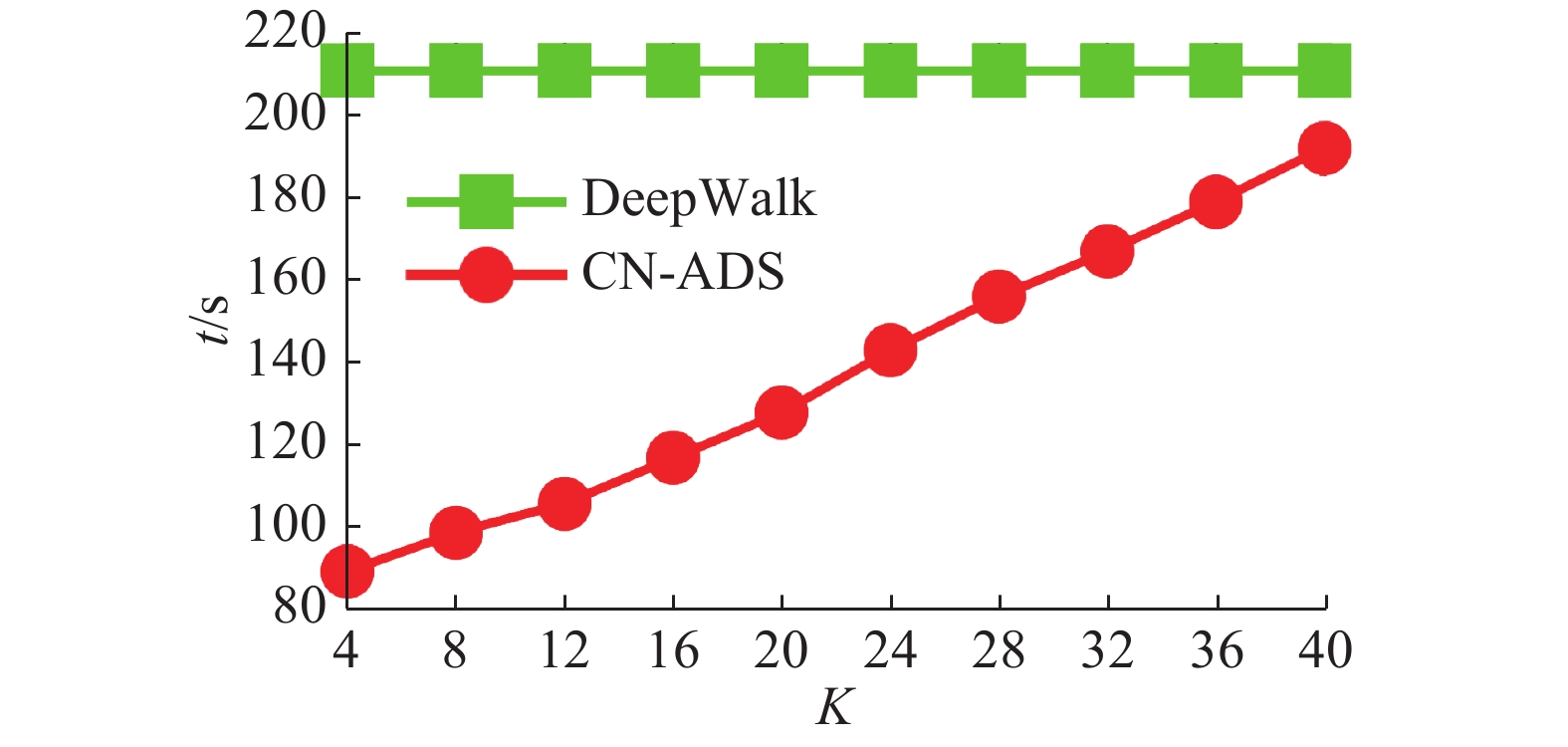Download: 图 8 PPI数据集上CN-ADS与DeepWalk的时间对比 Fig. 8 Time comparison of CN-ADS and DeepWalk on PPI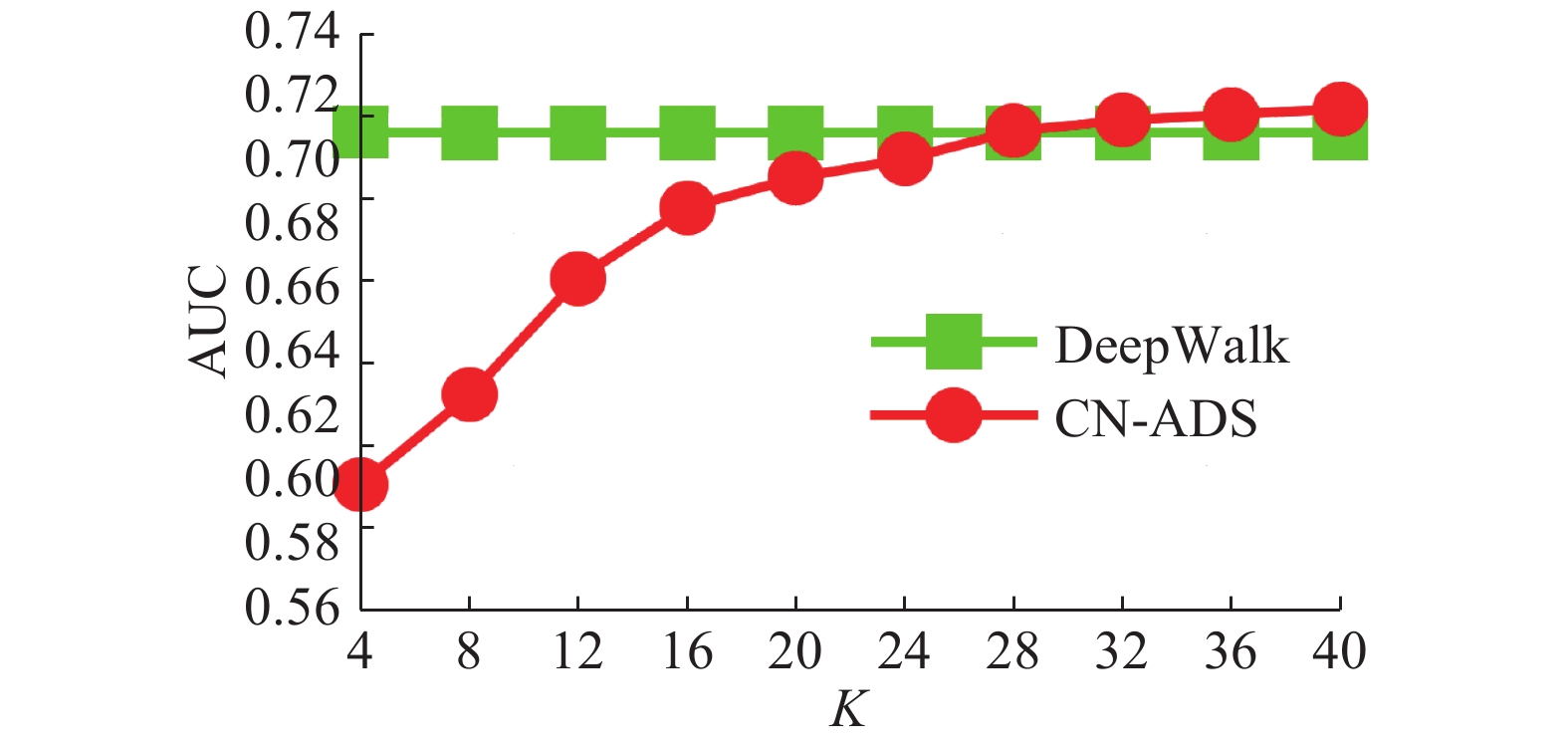Download: 图 9 PPI数据集上CN-ADS与DeepWalk的AUC对比 Fig. 9 AUC comparison of CN-ADS and DeepWalk on PPI
  吕琳媛. 复杂网络链路预测[J]. 电子科技大学学报, 2010, 39(5): 651-661. LYU Linyuan. Link prediction on complex networks[J]. Journal of University of Electronic Science and Technology of China, 2010, 39(5): 651-661. DOI:10.3969/j.issn.1001-0548.2010.05.002 (0)  CLAUSET A, MOORE C, NEWMAN M E J. Hierarchical structure and the prediction of missing links in networks[J]. Nature, 2008, 453(7191): 98-101. DOI:10.1038/nature06830 (0)  AIROLDI E M, BLEI D M, FIENBERG S E, et al. Mixed membership stochastic blockmodels[J]. Journal of machine learning research, 2008, 9: 1981-2014. (0)  YU Kai, CHU Wei, YU Shipeng, et al. Stochastic relational models for discriminative link prediction[C]//Proceedings of the 19th International Conference on Neural Information Processing Systems. Vancouver, Canada, 2006: 1553–1560. (0)  LIBEN-NOWELL D, KLEINBERG J. The link—prediction problem for social networks[J]. Journal of the association for information science and technology, 2007, 58(7): 1019-1031. (0)  ADAMIC L A, ADAR E. Friends and neighbors on the Web[J]. Social networks, 2003, 25(3): 211-230. DOI:10.1016/S0378-8733(03)00009-1 (0)  LYU Linyuan, JIN Cihang, ZHOU Tao. Similarity index based on local paths for link prediction of complex networks[J]. Physical review E, 2009, 80(4): 046122. DOI:10.1103/PhysRevE.80.046122 (0)  ZHOU Tao, Lü Linyuan, ZHANG Yicheng. Predicting missing links via local information[J]. The European physical journal B, 2009, 71(4): 623-630. DOI:10.1140/epjb/e2009-00335-8 (0)  KATZ L. A new status index derived from sociometric analysis[J]. Psychometrika, 1953, 18(1): 39-43. DOI:10.1007/BF02289026 (0)  LEICHT E A, HOLME P, NEWMAN M E J. Vertex similarity in networks[J]. Physical review E, 2006, 73: 026120. DOI:10.1103/PhysRevE.73.026120 (0)  KLEIN D J, RANDIĆ M. Resistance distance[J]. Journal of mathematical chemistry, 1993, 12(1): 81-95. DOI:10.1007/BF01164627 (0)  FOUSS F, PIROTTE A, RENDERS J M, et al. Random-walk computation of similarities between nodes of a graph with application to collaborative recommendation[J]. IEEE transactions on knowledge and data engineering, 2007, 19(3): 355-369. DOI:10.1109/TKDE.2007.46 (0)  BRIN S, PAGE L. The anatomy of a large-scale hypertextual Web search engine[J]. Computer networks and ISDN systems, 1998, 30(1-7): 107-117. DOI:10.1016/S0169-7552(98)00110-X (0)  JEH G, WIDOM J. SimRank: a measure of structural-context similarity[C]//Proceedings of the 8th ACM SIGKDD International Conference on Knowledge Discovery and Data Mining. Edmonton, Alberta, Canada, 2002: 538–543. (0)  饶君, 吴斌, 东昱晓. MapReduce环境下的并行复杂网络链路预测[J]. 软件学报, 2012, 23(12): 3175-3186. RAO Jun, WU Bin, DONG Yuxiao. Parallel link prediction in complex network using mapreduce[J]. Journal of software, 2012, 23(12): 3175-3186. (0)  COHEN E. All-distances sketches[M]//KAO M Y. Encyclopedia of Algorithms. New York: Springer, 2016: 2320-2334. (0)  BATAGELJ V, MRVAR A. Pajek datasets[EB/OL]. 2006 http://vlado.fmf.uni-lj.si/pub/networks/data/default.html. (0)  BU Dongbo, ZHAO Yi, CAI Lun, et al. Topological structure analysis of the protein–protein interaction network in budding yeast[J]. Nucleic acids research, 2003, 31(9): 2443-2450. (0)  WATTS D J, STROGATZ S H. Collective dynamics of ‘small-world’ networks[J]. Nature, 1998, 393(6684): 440-442. DOI:10.1038/30918 (0)  PEROZZI B, AL-RFOU R, SKIENA S. DeepWalk: online learning of social representations[C]//Proceedings of the 20th ACM SIGKDD International Conference on Knowledge Discovery and Data Mining. New York, USA, 2014: 701-710 (0)  BREITKREUTZ B J, STARK C, REGULY T, et al. The bioGRID interaction database: 2008 update[J]. Nucleic acids research, 2008, 36(S1): D637-D640. (0)